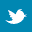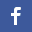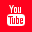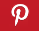Completely Solved C, C++ Programs Assignment.Quick Search Database Videos Tutorials Ebooks Forums FAQ Aboutus Household Industrial Manufacturing Service Shopping Transportation### C program to convert an Infix to a Postfix expression.

 Filed Under: C Assignments

Problem Statement
A C program to convert an Infix to a Postfix expression.

Theory:
Infix Expression: Any expression in the standard form like "2*3-4/5" is an Infix (Inorder) expression.
Postfix Expression: In the postfix notation the operators are written after the operands. The Postfix (Postorder) form of the above expression is "23*45/-".

Infix to Postfix Conversion:
Using infix notation one cannot tell the order in which operators should be applied by only looking at expression. Whenever an infix expression consists of more than one operators, the precedence rules(BODMAS) should be applied to decide that which operator (and operands associated with that operator) are evaluated first. As compared to postfix notation, which is much more easy to work with or evaluate. In a postfix expression operands appear before the operators, there is no need for operator precedence and other rules. As soon as an operator appears in the postfix expression during scanning of postfix expression the topmost operands are popped off and are calculated applying the encountered operator.

Algorithm:
POLISH(Q,P)
Suppose Q is an arithmetic expression written in infix notation, this algorithm finds the equivalent postfix expression.

Step1: Push "(" onto STACK, and add ")" to the end of Q.

Step2: Scan Q from left to right and repeat steps 3 to 6 for each element of Q until the stack is empty.
Step 3: If an operand is encountered add it to P.

Step 4: If a left parenthesis is encountered push it onto the stack

Step 5: If an operator is encountered, then
(a) Repeatedly pop from STACK and add to P each operator (on top of the STACK) which has same precedence as or
higher precedence than the operator encountered
(b) Add the encountered operator to the stack.
[End of IF structure]

Step6: If a right parenthesis is encountered, then
(a) repeatedly pop from the stack and add to P each operator (on top of the STACK) until a left parenthesis is encountered.
(b) Remove the left parenthesis. [do not add left parenthesis to P]
[End of IF structure]
[End of step 2 loop]

Step7: Exit.

Program Listing:
#include<stdio.h>
#include<string.h>
enum{False,True};
#define sub 1
#define mul 2
#define div 2
#define exp 3
int top =0;
void push(char[],char);
char pop(char[]);
int stack_empty();
void main()
{
char s;
void i2p(char[]);
printf("Enter the Infix expression: ");
scanf("%s",s);
printf("nThe Postfix expression is: ");
i2p(s);

}
int get_type(char symbol)
{
switch(symbol)
{
case '(':
return 1;
case ')':
return 2;
case '+':
case '-':
case '*':
case '/':
case '^':
return 4;
default:
return 3;
}
}

int get_precedence(char symbol)
{
switch(symbol)
{
case '+':
case '-':
return sub;
case '*':
return mul;
case'/':
return div;
case'^':
return exp;
case '(':
return 0;
deault:
return;
}
}

void i2p(char infix[])
{

int i=0,p=0,len,type,prec;
char next,stack,postfix;
int get_type(char);
int get_precedence(char);
len=strlen(infix);

while(i<len)
{
type=get_type(infix[i]);
switch(type)
{
case 1:
push(stack,infix[i]);
break;
case 2:
while((next=pop(stack))!='(')
postfix[p++]=next;
break;
case 3:
postfix[p++]=infix[i];
break;
case 4:
prec=get_precedence(infix[i]);
while(stack_empty()==False)
{
if(prec<=get_precedence(stack[top-1]))
postfix[p++]=pop(stack);
else
break;
}
push(stack,infix[i]);
break;
}
i++;
}
while(stack_empty()==False)
postfix[p++]=pop(stack);
postfix[p]='�';
printf("%sn",postfix);
}

void push(char stack[],char data)
{
stack[top]=data;
top++;
}

char pop(char stack[])
{
char data;
top--;
data=stack[top];
return data;
}

int stack_empty()
{
if(top==0)
return True;
else
return False;
}

Output:
SET1
Enter the Infix expression: 7-(2*3+5)*(8-4/2)

The Postfix expression is: 723*5+842/-*-

SET2
Enter the infix expression: A*(B+D)/E-F*(G+H/K)

The Postfix expression is: ABD+*E/FGHK/+*-

Discussion :
• It is advantageous to use postfix notation. As operands appear before the operators, there is no need for operator precedence and other rules.

• Here the stack is implemented using array. As the size of array is declared before the start of the operation, it is not too efficient with respect to memory utilization.

• The same program can be done using linked list which is more efficient than array in terms of memory utilization.Back to main directory:Software Practical